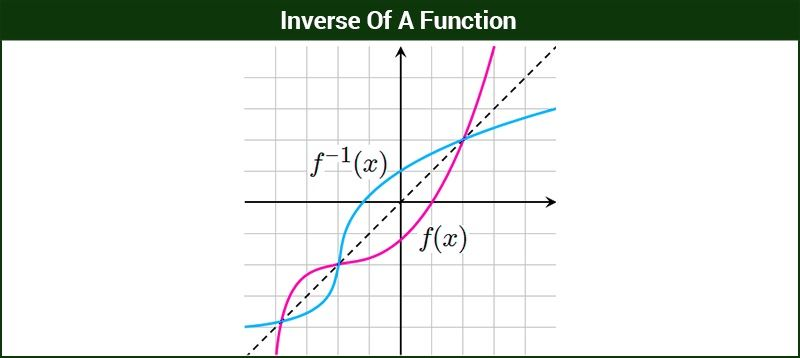Inverse Of A Function

A function $f: A \rightarrow B$ is said to have an inverse if there exists a function $h : B \rightarrow A$ such that $hof = I_A$ and $foh = I_B$. The function h is called the inverse of f.Inverse of a function f is denoted by $f^{-1}$

Conditions for the function ‘f’ to be invertible are

1) f must be a one-one function

2) f must be an onto function.

Let us go through few problems to understand the concept better.

Example 1: Consider $f:{a, e, i, o, u} \rightarrow {a, b, c, d}$  with $f$ = ${(a, a)(e, b)(i, c)(o, d)(u, d)}$. Check whether this function has an inverse.

Solution: The function is not one-one as two elements of the domain (o,u) are mapped to the same element (d) in the co-domain.

The function is onto as all the elements of the co-domain have pre-images (Note that if a function is not one-one, a check for onto is not necessary).

The function f is not invertible as f is not one-one.

Example 2: Find the inverse of f if $f: N \rightarrow R$ defined as $f(x)$ = $4x^2 + 20x + 25$ and $f : N \rightarrow A$  where A is the range of f, is invertible.

Solution:  Consider arbitrary element $a ∈ A$.

$a$ = $4x^2 + 20x + 25$ for some x in N

$a$ = $(2x + 5)^2$

$2x$ = $\pm \sqrt{a} – 5$

When $-\sqrt{a}$ is considered, x becomes a negative number and hence is not a Natural number anymore. So,
$2x$ = $\sqrt{a} – 5$

$x$ = $\frac{(\sqrt{a} – 5)}{2}$

$f^{-1}$ = $\frac{(\sqrt{a} – 5)}{2}$

$f^{-1}(x)$ = $\frac{(\sqrt{x} – 5)}{2}$

Example 3: Does an identity function with domain and co-domain as natural numbers have an inverse?

Solution: Consider the identity function f(x) = x

Identity function $f: N \rightarrow N$  is one-one and onto.

Therefore, f has an inverse.

Consider $a ∈ N$ (co-domain)

$a$ = $x$

$f^{-1}$ = $a$

$f^{-1}(x)$ =$x$

Example 4: Consider A = {1, 4, 9, 16, 25, 36}. Determine whether the function $f: A \rightarrow A$ defined by f = {(1,1), (4, 9), (9, 16), (16, 4), (25, 36), (36, 25)} has an inverse.

Solution:  The function $f: A \rightarrow A$ is one-one and onto. Therefore, the function has an inverse.

$f^{-1}(1)$ = $1$

$f^{-1}(9)$ = $4$

$f^{-1}(16)$ = $9$

$f^{-1}(4)$ = $16$

$f^{-1}(36)$ =$25$

$f^{-1}(25)$ = $36$<